Name:    2nd Grade Geometry - Reason with shapes and their attrributes, Standard 2 Pre Test Partition a rectangle into rows and columns of same-size squares and count to find the total number of them.

 Numeric Response 1.How many of these squares are colored green? 2.How many of these squares are colored orange? 3.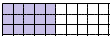How many of these squares are colored purple? 4.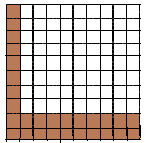How many of these squares are colored brown? 5.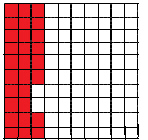How many of these squares are colored red? 6.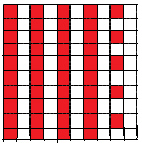How many of these squares are colored red? 7.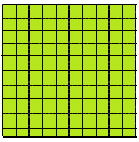How many of these squares are colored green?